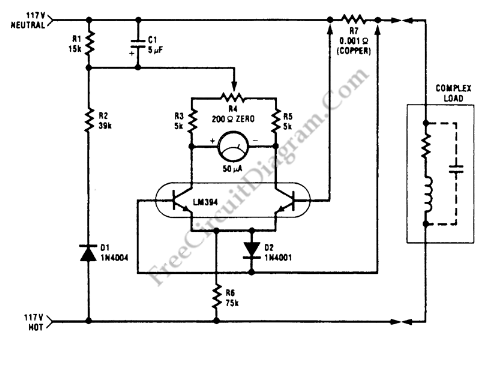# 1 KW Power (Watt) MeterThis watt-meter circuit has measurement range up to 1-KW. This circuit can give the complete (X)(Y) function although uses only one transistor. Actually, this circuit is used for 117 Vac±50 Vac operation. For lower or lower voltage, this circuit can be modified easily. This circuit only measure power on negative cycles. The advantages of this circuit is this circuit does not need external power supply. This circuit measures true power that is delivered to the load. Here is the schematic diagram of the  circuit:At idle section, this circuit draw only 0.5W. This circuit has load current-sensing voltage of 10mV and load voltage loss of 0.01%. For linear loads, Rejection of reactive load currents is better than 100:1. When using a 50-μA meter movement, the nonlinearity of this circuit is about 1% full scale. Copper shunt can be used to give correct gain due to temperature. [Circuit’s schematic diagram source: seekic.com]